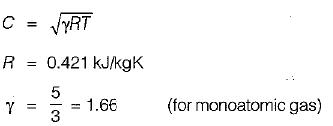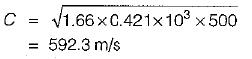Courses

# Test: Flow of Compressible Fluids - 2

## 10 Questions MCQ Test Topicwise Question Bank for Mechanical Engineering | Test: Flow of Compressible Fluids - 2

Description
This mock test of Test: Flow of Compressible Fluids - 2 for Mechanical Engineering helps you for every Mechanical Engineering entrance exam. This contains 10 Multiple Choice Questions for Mechanical Engineering Test: Flow of Compressible Fluids - 2 (mcq) to study with solutions a complete question bank. The solved questions answers in this Test: Flow of Compressible Fluids - 2 quiz give you a good mix of easy questions and tough questions. Mechanical Engineering students definitely take this Test: Flow of Compressible Fluids - 2 exercise for a better result in the exam. You can find other Test: Flow of Compressible Fluids - 2 extra questions, long questions & short questions for Mechanical Engineering on EduRev as well by searching above.
QUESTION: 1

Solution:
QUESTION: 2

### Across a normal shock

Solution: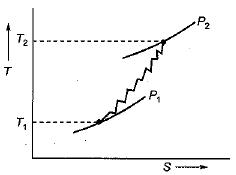Hence during the normal shock wave static pressure and static temperature rise takes place.

QUESTION: 3

### Consider the curves in the sketch shown below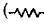indicates normal shock)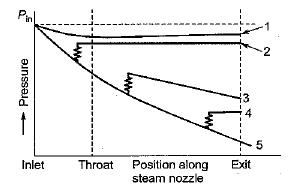Out of these curves, those which are not correctly drawn will include

Solution:

Since shock will not be experienced at converging portion hence plot (2) is wrong. During the shock flow velocity decreases and pressure rise takes place but in figure (3) pressure in decreasing hence it is wrong.

QUESTION: 4

Consider the following statements:
A tube, with the section diverging in the direction of flow, can be used as
1. Supersonic nozzle
2. Subsonic nozzle
3. Supersonic diffuser
4. Subsonic diffuser

Select the correct answer using the codes given below:

Solution: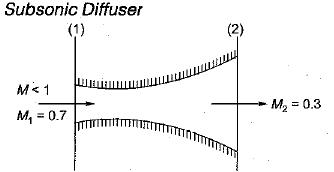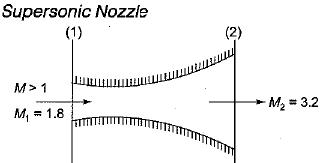QUESTION: 5

Match List-I with List-ll and select the correct answer using the codes given below the lists: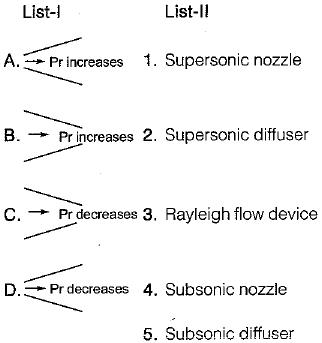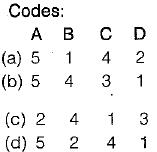Solution:
QUESTION: 6

An aeroplane is cruising at a speed of 800 kmph at an altitude, where the air temperature is 0°C. The flight Mach number at this speed is nearly,

Solution: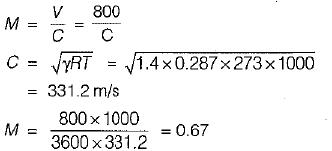QUESTION: 7

For a compressible fluid, sonic velocity is

Solution:
QUESTION: 8

Which one of the following properties drops across a normal shock?

Solution:
QUESTION: 9

The flight Mach number of an aircraft flying at an altitude where the temperature is -48°C is 0.7. The speed of the aircraft is (Take γ = 1.4, 77= 287 J/kgK)

Solution:
QUESTION: 10

What is the speed of sound in Neon gas at temperature of 500 K (Gas constant of Neon is 0.4210 kJ/kgK)?

Solution: